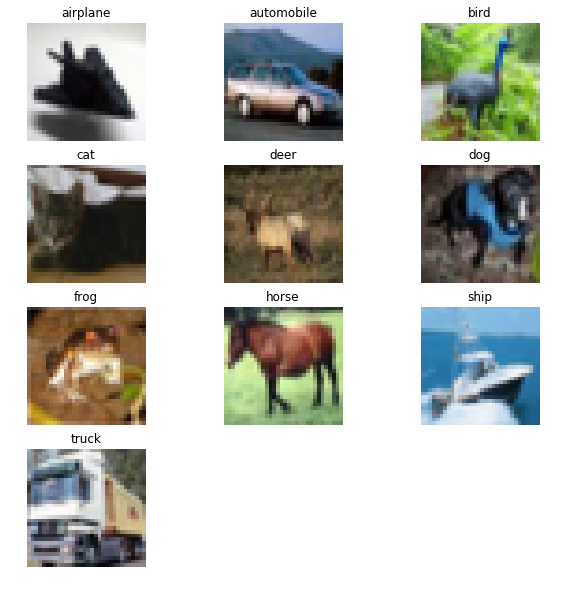# Exercise 7¶

Data Preparation

We will train our network on the CIFAR10 dataset, which contains 50,000 32x32 color training images, labeled over 10 categories, and 10,000 test images.

As this dataset is also included in Keras datasets, we just ask the keras.datasets module for the dataset.

Training and test images are normalized to lie in the $\left[0,1\right]$ interval.

In :
from keras.datasets import cifar10
from keras.utils import np_utils
import pandas as pd
import numpy as np
import matplotlib.pyplot as plt
import matplotlib.image as mpimg
%matplotlib inline

/home/al/anaconda3/lib/python3.6/site-packages/h5py/__init__.py:36: FutureWarning: Conversion of the second argument of issubdtype from float to np.floating is deprecated. In future, it will be treated as np.float64 == np.dtype(float).type.
from ._conv import register_converters as _register_converters
Using TensorFlow backend.

In :
# Normal option with full database
# (X_train, y_train), (X_test, y_test) = cifar10.load_data()  # File is 160MB

In :
# Only the first training batch and testing  # File is 58MB
import tarfile
from six.moves import cPickle

# Use local copy of the cifar-10 database
data_tar = tarfile.open('../datasets/cifar-10-python_trainingbatch1_test.tar.gz')

def extract_xy(member, data_tar):
d = cPickle.load(data_tar.extractfile(member), encoding='bytes')
d_decoded = {}
for k, v in d.items():
d_decoded[k.decode('utf8')] = v
d = d_decoded
X = d['data']
X = X.reshape(X.shape, 3, 32, 32)
y = d['labels']
return X.transpose(0, 2, 3, 1), np.reshape(y, (len(y), 1))

X_train, y_train = extract_xy('cifar-10-batches-py/data_batch_1', data_tar)
X_test, y_test = extract_xy('cifar-10-batches-py/test_batch', data_tar)

In :
X_train.shape, X_test.shape

Out:
((10000, 32, 32, 3), (10000, 32, 32, 3))
In :
nb_classes = y_train.max() + 1
Y_train = np_utils.to_categorical(y_train, nb_classes)
Y_test = np_utils.to_categorical(y_test, nb_classes)
X_train = X_train.astype("float32")
X_test = X_test.astype("float32")
X_train /= 255
X_test /= 255

In :
labels = ['airplane', 'automobile','bird', 'cat', 'deer', 'dog', 'frog', 'horse', 'ship','truck']

In :
X_train.shape

Out:
(32, 32, 3)
In :
y_train

Out:
array()
In :
Y_train

Out:
array([0., 0., 0., 0., 0., 0., 1., 0., 0., 0.], dtype=float32)
In :
# Select one image per y
images = [np.nonzero(Y_train[:,i] == 1) for i in range(nb_classes)]

# Plot all images
fig, ax = plt.subplots(4,3, figsize=(10,10))
ax = ax.reshape(12)
for i in range(nb_classes):
ax[i].imshow(X_train[images[i]])
ax[i].set_title(labels[y_train[images[i]]])
ax[i].axis('off')

ax[-1].axis('off')
ax[-2].axis('off')
plt.show()# Exercise 08.1¶

Train a Softmax regression using keras Optimized using rmsprop using as loss categorical_crossentropy

Hints:

• test with two iterations then try more.
• learning can be ajusted

Evaluate the performance using the testing set (aprox 31% with 50 epochs)

In :
from keras.models import Sequential
from keras.layers import Dense, Dropout, Activation, Flatten
from keras.layers import Conv2D, MaxPooling2D
from livelossplot import PlotLossesKeras


# Exercise 08.2¶

Train a Deep Neural Network with the following architecture:

• Input = Image (32, 32, 3)
• Conv2d(32, (3,3), padding='same')
• Relu Activation
• MaxPooling2D (2,2)
• Dropout(0.5)
• Flattern
• Dense(10, Softmax)

Optimized using rmsprop using as loss categorical_crossentropy

Hints:

• test with two iterations then try more.
• learning can be ajusted

Evaluate the performance using the testing set (aprox 55% with 50 epochs)

# Exercise 08.3¶

Use the last layer of VGG16 as the input to a neural network with softmax output

• Input = Image (32, 32, 3)
• VGG16
• Flattern
• Dense(512, Softmax)
• Dropout(0.5)
• Dense(10, Softmax)

Optimized using rmsprop using as loss categorical_crossentropy

Hints:

• test with two iterations then try more.
• learning can be ajusted

Evaluate the performance using the testing set (aprox 57% with 50 epochs)

In :
from keras.applications.vgg16 import VGG16
model_vgg16 = VGG16(weights='imagenet', include_top=False)


# Exercise *08.4 (Bonus)¶

Test a different pre-train algorithm (https://keras.io/applications/#usage-examples-for-image-classification-models)